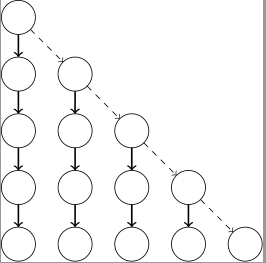## $k$ 祖先问题

$k$ 祖先问题（Level Ancestor Problem）是静态树的重要问题之一。给定一棵树和若干个询问 $(u, k)$，要求结点 $u$ 的第 $k​$ 个祖先结点。

## 长链剖分

### $O(n)-O(\log n)$ 算法

1. 轻儿子的深度之和 $\le n$。将轻儿子的深度附着在这个轻儿子的重链上，显而易见每一条重链只会提供 $1$ 的贡献，因此轻儿子深度之和 $\le n$。

2. 结点 $u$ 的 $k$ 祖先 $g$ 所在的重链长度至少是 $k$。若不然，$g\to u$ 构成了一条更高的链。

• 对于询问 $(u, k)​$，若 $k​$ 祖先仍在重链和其上部之内，查表得到 $k​$ 祖先
• 否则，跳到重链上部的顶端，并更新 $u$ 和 $k$### $O(n\log n)-O(1)$ 算法

• 用 $O(n\log n)$ 的时间复杂度预处理倍增表 $f[i][u]$
• 预处理长链剖分，并计算每条长链的上端
• 对于询问 $(u, k)$，设 $k$ 二进制下的最高位是 $d$，跳到 $f[d][u]$。根据上面的性质 $2$，一定可以通过在长链及其上端查表得到 $k$ 祖先。

### 线性时间优化

• 在 dfs 处理结点 $nd$ 的过程中，如果子树大小小于 $b$ 则不处理，否则将 $nd$ 选为关键点，并删去整个子树。

Note：1中给出了一种更简单的分块方法。

## 参考文献

1. MIT 6.851, Advanced Data Structure, Static tree(1)